Pulkit Sharma — December 26, 2018

## Introduction

If you had to pick one deep learning technique for computer vision from the plethora of options out there, which one would you go for? For a lot of folks, including myself, convolutional neural network is the default answer.

But what is a convolutional neural network and why has it suddenly become so popular? Well, that’s what we’ll find out in this article! CNNs have become the go-to method for solving any image data challenge. Their use is being extended to video analytics as well but we’ll keep the scope to image processing for now. Any data that has spatial relationships is ripe for applying CNN – let’s just keep that in mind for now.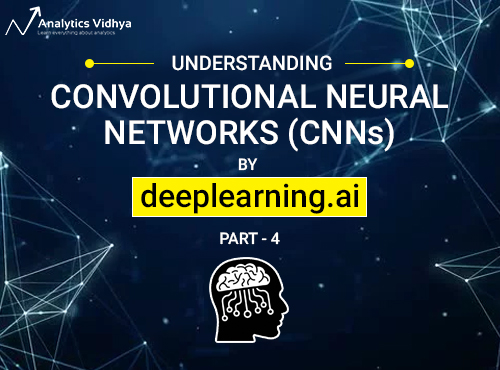In the previous articles in this series, we learned the key to deep learning – understanding how neural networks work. We saw how using deep neural networks on very large images increases the computation and memory cost. To combat this obstacle, we will see how convolutions and convolutional neural networks help us to bring down these factors and generate better results.

So welcome to part 3 of our deeplearning.ai course series (deep learning specialization) taught by the great Andrew Ng. In addition to exploring how a convolutional neural network (ConvNet) works, we’ll also look at different architectures of a ConvNet and how we can build an object detection model using YOLO. Finally, we’ll tie our learnings together to understand where we can apply these concepts in real-life applications (like facial recognition and neural style transfer).

I highly recommend going through the first two parts before diving into this guide:

1. Course Structure
2. Course #4: Convolutional Neural Networks
1. Module 1: Foundations of Convolutional Neural Networks
2. Module 2: Deep Convolutional Models: Case Studies
1. Case Studies
2. Practical Advice for using ConvNets
3. Module 3: Object Detection
4. Module 4: Special Applications: Face Recognition & Neural Style Transfer

## Course Structure

The previous articles of this series covered the basics of deep learning and neural networks. We also learned how to improve the performance of a deep neural network using techniques like hyperparameter tuning, regularization and optimization.

So where to next? Let’s turn our focus to the concept of Convolutional Neural Networks. Course #4 of the deep learning specialization is divided into 4 modules:

1. In module 1, we will understand the convolution and pooling operations and will also look at a simple Convolutional Network example
2. In module 2, we will look at some practical tricks and methods used in deep CNNs through the lens of multiple case studies. We will also learn a few practical concepts like transfer learning, data augmentation, etc.
3. Module 3 will cover the concept of object detection. We will discuss the popular YOLO algorithm and different techniques used in YOLO for object detection
4. Finally, in module 4, we will briefly discuss how face recognition and neural style transfer work. This is a very interesting module so keep your learning hats on till the end

## Course 4: Convolutional Neural Network

Ready? Good, because we are diving straight into module 1!

## Week 1: Foundations of Convolutional Neural Networks

The objectives behind the first module of the course 4 are:

• To understand the convolution operation
• To understand the pooling operation
• Remembering the vocabulary used in convolutional neural networks (padding, stride, filter, etc.)
• Building a convolutional neural network for multi-class classification in images

### Computer Vision

Some of the computer vision problems which we will be solving in this article are:

1. Image classification
2. Object detection
3. Neural style transfer

One major problem with computer vision problems is that the input data can get really big. Suppose an image is of the size 68 X 68 X 3. The input feature dimension then becomes 12,288. This will be even bigger if we have larger images (say, of size 720 X 720 X 3). Now, if we pass such a big input to a neural network, the number of parameters will swell up to a HUGE number (depending on the number of hidden layers and hidden units). This will result in more computational and memory requirements – not something most of us can deal with.

### Edge Detection Example

In the previous article, we saw that the early layers of a neural network detect edges from an image. Deeper layers might be able to detect the cause of the objects and even more deeper layers might detect the cause of complete objects (like a person’s face).

In this section, we will focus on how the edges can be detected from an image. Suppose we are given the below image:As you can see, there are many vertical and horizontal edges in the image. The first thing to do is to detect these edges: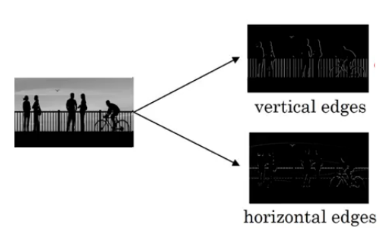But how do we detect these edges? To illustrate this, let’s take a 6 X 6 grayscale image (i.e. only one channel):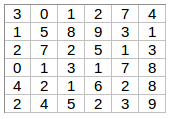Next, we convolve this 6 X 6 matrix with a 3 X 3 filter: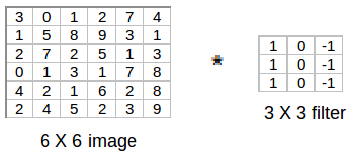After the convolution, we will get a 4 X 4 image. The first element of the 4 X 4 matrix will be calculated as: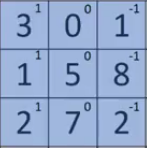So, we take the first 3 X 3 matrix from the 6 X 6 image and multiply it with the filter. Now, the first element of the 4 X 4 output will be the sum of the element-wise product of these values, i.e. 3*1 + 0 + 1*-1 + 1*1 + 5*0 + 8*-1 + 2*1 + 7*0 + 2*-1 = -5. To calculate the second element of the 4 X 4 output, we will shift our filter one step towards the right and again get the sum of the element-wise product: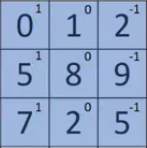Similarly, we will convolve over the entire image and get a 4 X 4 output: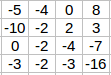So, convolving a 6 X 6 input with a 3 X 3 filter gave us an output of 4 X 4. Consider one more example: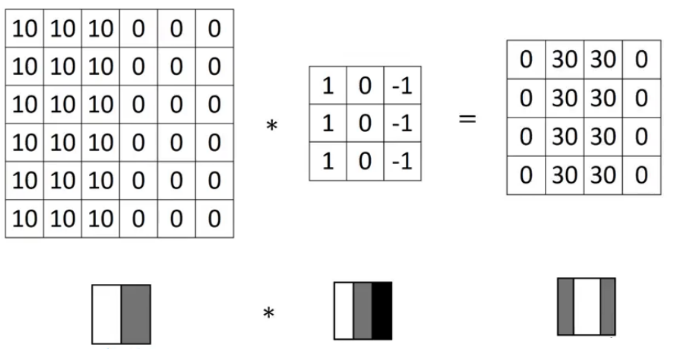Note: Higher pixel values represent the brighter portion of the image and the lower pixel values represent the darker portions. This is how we can detect a vertical edge in an image.

### More Edge Detection

The type of filter that we choose helps to detect the vertical or horizontal edges. We can use the following filters to detect different edges: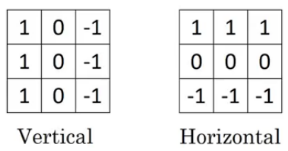Some of the commonly used filters are: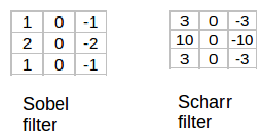The Sobel filter puts a little bit more weight on the central pixels. Instead of using these filters, we can create our own as well and treat them as a parameter which the model will learn using backpropagation.

We have seen that convolving an input of 6 X 6 dimension with a 3 X 3 filter results in 4 X 4 output. We can generalize it and say that if the input is n X n and the filter size is f X f, then the output size will be (n-f+1) X (n-f+1):

• Input: n X n
• Filter size: f X f
• Output: (n-f+1) X (n-f+1)

There are primarily two disadvantages here:

1. Every time we apply a convolutional operation, the size of the image shrinks
2. Pixels present in the corner of the image are used only a few number of times during convolution as compared to the central pixels. Hence, we do not focus too much on the corners since that can lead to information loss

To overcome these issues, we can pad the image with an additional border, i.e., we add one pixel all around the edges. This means that the input will be an 8 X 8 matrix (instead of a 6 X 6 matrix). Applying convolution of 3 X 3 on it will result in a 6 X 6 matrix which is the original shape of the image. This is where padding comes to the fore:

• Input: n X n
• Filter size: f X f
• Output: (n+2p-f+1) X (n+2p-f+1)

There are two common choices for padding:

1. Valid: It means no padding. If we are using valid padding, the output will be (n-f+1) X (n-f+1)
2. Same: Here, we apply padding so that the output size is the same as the input size, i.e.,
n+2p-f+1 = n
So, p = (f-1)/2

We now know how to use padded convolution. This way we don’t lose a lot of information and the image does not shrink either. Next, we will look at how to implement strided convolutions.

### Strided Convolutions

Suppose we choose a stride of 2. So, while convoluting through the image, we will take two steps – both in the horizontal and vertical directions separately. The dimensions for stride s will be:

• Input: n X n
• Stride: s
• Filter size: f X f
• Output: [(n+2p-f)/s+1] X [(n+2p-f)/s+1]

Stride helps to reduce the size of the image, a particularly useful feature.

### Convolutions Over Volume

Suppose, instead of a 2-D image, we have a 3-D input image of shape 6 X 6 X 3. How will we apply convolution on this image? We will use a 3 X 3 X 3 filter instead of a 3 X 3 filter. Let’s look at an example:

• Input: 6 X 6 X 3
• Filter: 3 X 3 X 3

The dimensions above represent the height, width and channels in the input and filter. Keep in mind that the number of channels in the input and filter should be same. This will result in an output of 4 X 4. Let’s understand it visually: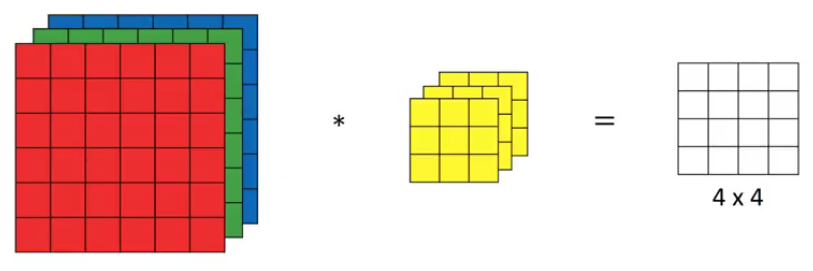Since there are three channels in the input, the filter will consequently also have three channels. After convolution, the output shape is a 4 X 4 matrix. So, the first element of the output is the sum of the element-wise product of the first 27 values from the input (9 values from each channel) and the 27 values from the filter. After that we convolve over the entire image.

Instead of using just a single filter, we can use multiple filters as well. How do we do that? Let’s say the first filter will detect vertical edges and the second filter will detect horizontal edges from the image. If we use multiple filters, the output dimension will change. So, instead of having a 4 X 4 output as in the above example, we would have a 4 X 4 X 2 output (if we have used 2 filters):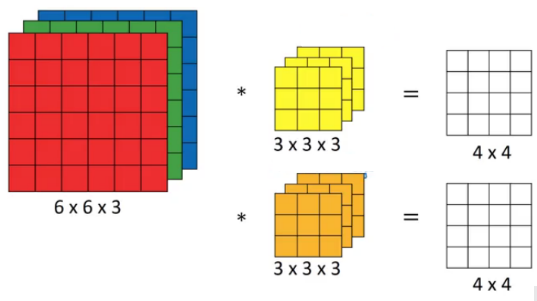Generalized dimensions can be given as:

• Input: n X n X nc
• Filter: f X f X nc
• Stride: s
• Output: [(n+2p-f)/s+1] X [(n+2p-f)/s+1] X nc

Here, nc is the number of channels in the input and filter, while nc’ is the number of filters.

### One Layer of a Convolutional Network

Once we get an output after convolving over the entire image using a filter, we add a bias term to those outputs and finally apply an activation function to generate activations. This is one layer of a convolutional network. Recall that the equation for one forward pass is given by:

z = w*a + b
a = g(z)

In our case, input (6 X 6 X 3) is aand filters (3 X 3 X 3) are the weights w. These activations from layer 1 act as the input for layer 2, and so on. Clearly, the number of parameters in case of convolutional neural networks is independent of the size of the image. It essentially depends on the filter size. Suppose we have 10 filters, each of shape 3 X 3 X 3. What will be the number of parameters in that layer? Let’s try to solve this:

• Number of parameters for each filter = 3*3*3 = 27
• There will be a bias term for each filter, so total parameters per filter = 28
• As there are 10 filters, the total parameters for that layer = 28*10 = 280

No matter how big the image is, the parameters only depend on the filter size. Awesome, isn’t it? Let’s have a look at the summary of notations for a convolution layer:

• f[l] = filter size
• s[l] = stride
• n[c][l] = number of filters

Let’s combine all the concepts we have learned so far and look at a convolutional network example.

### Simple Convolutional Network Example

This is how a typical convolutional network looks like: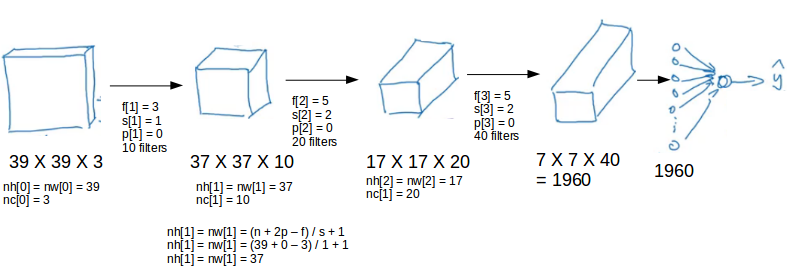We take an input image (size = 39 X 39 X 3 in our case), convolve it with 10 filters of size 3 X 3, and take the stride as 1 and no padding. This will give us an output of 37 X 37 X 10. We convolve this output further and get an output of 7 X 7 X 40 as shown above. Finally, we take all these numbers (7 X 7 X 40 = 1960), unroll them into a large vector, and pass them to a classifier that will make predictions. This is a microcosm of how a convolutional network works.

There are a number of hyperparameters that we can tweak while building a convolutional network. These include the number of filters, size of filters, stride to be used, padding, etc. We will look at each of these in detail later in this article. Just keep in mind that as we go deeper into the network, the size of the image shrinks whereas the number of channels usually increases.

In a convolutional network (ConvNet), there are basically three types of layers:

1. Convolution layer
2. Pooling layer
3. Fully connected layer

Let’s understand the pooling layer in the next section.

### Pooling Layers

Pooling layers are generally used to reduce the size of the inputs and hence speed up the computation. Consider a 4 X 4 matrix as shown below: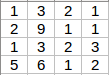Applying max pooling on this matrix will result in a 2 X 2 output: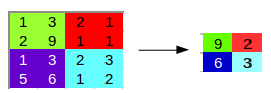For every consecutive 2 X 2 block, we take the max number. Here, we have applied a filter of size 2 and a stride of 2. These are the hyperparameters for the pooling layer. Apart from max pooling, we can also apply average pooling where, instead of taking the max of the numbers, we take their average. In summary, the hyperparameters for a pooling layer are:

1. Filter size
2. Stride
3. Max or average pooling

If the input of the pooling layer is nh X nw X nc, then the output will be [{(nh – f) / s + 1} X {(nw – f) / s + 1} X nc].

### CNN Example

We’ll take things up a notch now. Let’s look at how a convolution neural network with convolutional and pooling layer works. Suppose we have an input of shape 32 X 32 X 3: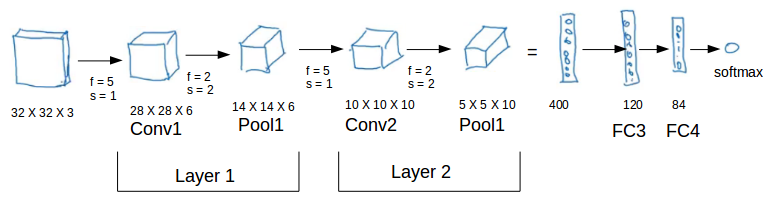There are a combination of convolution and pooling layers at the beginning, a few fully connected layers at the end and finally a softmax classifier to classify the input into various categories. There are a lot of hyperparameters in this network which we have to specify as well.

Generally, we take the set of hyperparameters which have been used in proven research and they end up doing well. As seen in the above example, the height and width of the input shrinks as we go deeper into the network (from 32 X 32 to 5 X 5) and the number of channels increases (from 3 to 10).

All of these concepts and techniques bring up a very fundamental question – why convolutions? Why not something else?

### Why Convolutions?

There are primarily two major advantages of using convolutional layers over using just fully connected layers:

1. Parameter sharing
2. Sparsity of connections

Consider the below example: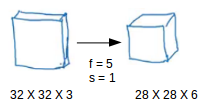If we would have used just the fully connected layer, the number of parameters would be = 32*32*3*28*28*6, which is nearly equal to 14 million! Makes no sense, right?

If we see the number of parameters in case of a convolutional layer, it will be = (5*5 + 1) * 6 (if there are 6 filters), which is equal to 156. Convolutional layers reduce the number of parameters and speed up the training of the model significantly.

In convolutions, we share the parameters while convolving through the input. The intuition behind this is that a feature detector, which is helpful in one part of the image, is probably also useful in another part of the image. So a single filter is convolved over the entire input and hence the parameters are shared.

The second advantage of convolution is the sparsity of connections. For each layer, each output value depends on a small number of inputs, instead of taking into account all the inputs.

## Module 2: Deep Convolutional Models: Case Studies

The objective behind the second module of course 4 are:

• To understand multiple foundation papers of convolutional neural networks
• To analyze the dimensionality reduction of a volume in a very deep network
• Understanding and implementing a residual network
• Building a deep neural network using Keras
• Implementing a skip-connection in your network
• Cloning a repository from GitHub and using transfer learning

### Classic Networks

In this section, we will look at the following popular networks:

1. LeNet-5
2. AlexNet
3. VGG

We will also see how ResNet works and finally go through a case study of an inception neural network.

LeNet-5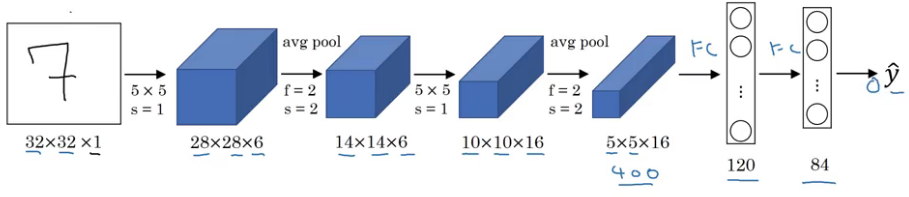It takes a grayscale image as input. Once we pass it through a combination of convolution and pooling layers, the output will be passed through fully connected layers and classified into corresponding classes. The total number of parameters in LeNet-5 are:

• Parameters: 60k
• Layers flow: Conv -> Pool -> Conv -> Pool -> FC -> FC -> Output
• Activation functions: Sigmoid/tanh and ReLu

AlexNet

An illustrated summary of AlexNet is given below: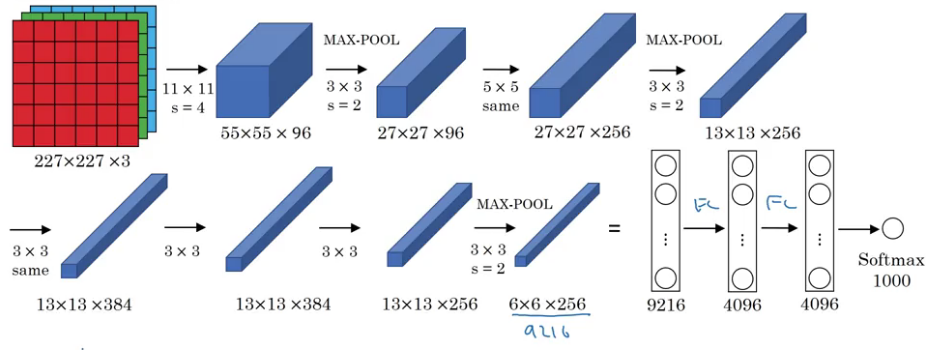This network is similar to LeNet-5 with just more convolution and pooling layers:

• Parameters: 60 million
• Activation function: ReLu

VGG-16

The underlying idea behind VGG-16 was to use a much simpler network where the focus is on having convolution layers that have 3 X 3 filters with a stride of 1 (and always using the same padding). The max pool layer is used after each convolution layer with a filter size of 2 and a stride of 2. Let’s look at the architecture of VGG-16: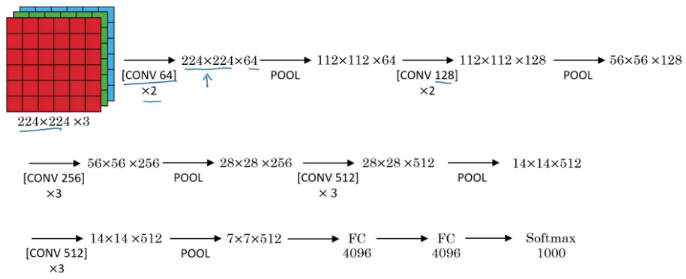As it is a bigger network, the number of parameters are also more.

• Parameters: 138 million

These are three classic architectures. Next, we’ll look at more advanced architecture starting with ResNet.

### ResNet

Training very deep networks can lead to problems like vanishing and exploding gradients. How do we deal with these issues? We can use skip connections where we take activations from one layer and feed it to another layer that is even more deeper in the network. There are residual blocks in ResNet which help in training deeper networks.

Residual Blocks

The general flow to calculate activations from different layers can be given as: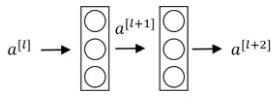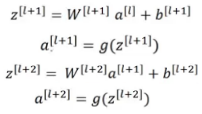This is how we calculate the activations a[l+2] using the activations a[l] and then a[l+1]. a[l] needs to go through all these steps to generate a[l+2]: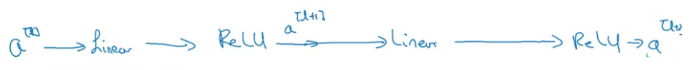In a residual network, we make a change in this path. We take the activations a[l] and pass them directly to the second layer: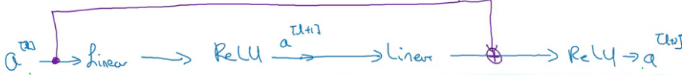So, the activations a[l+2] will be:

a[l+2] = g(z[l+2] + a[l])

The residual network can be shown as: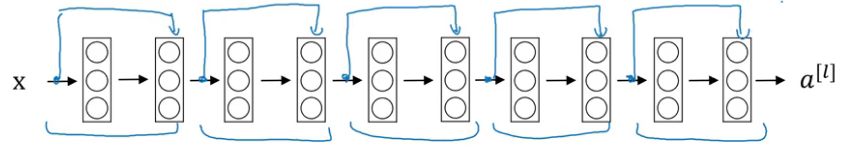The benefit of training a residual network is that even if we train deeper networks, the training error does not increase. Whereas in case of a plain network, the training error first decreases as we train a deeper network and then starts to rapidly increase: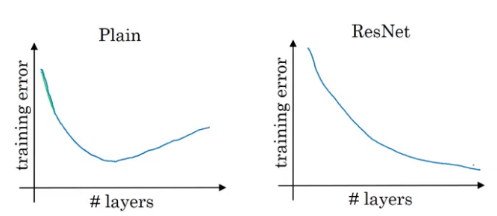We now have an overview of how ResNet works. But why does it perform so well? Let’s find out!

### Why ResNets Work?

In order to make a good model, we first have to make sure that it’s performance on the training data is good. That’s the first test and there really is no point in moving forward if our model fails here. We have seen earlier that training deeper networks using a plain network increases the training error after a point of time. But while training a residual network, this isn’t the case. Even when we build a deeper residual network, the training error generally does not increase.

The equation to calculate activation using a residual block is given by:

a[l+2] = g(z[l+2] + a[l])
a[l+2] = g(w[l+2] * a[l+1] + b[l+2] + a[l])

Now, say w[l+2] = 0 and the  bias b[l+2] is also 0, then:

a[l+2] = g(a[l])

It is fairly easy to calculate a[l+2] knowing just the value of a[l]. As per the research paper, ResNet is given by: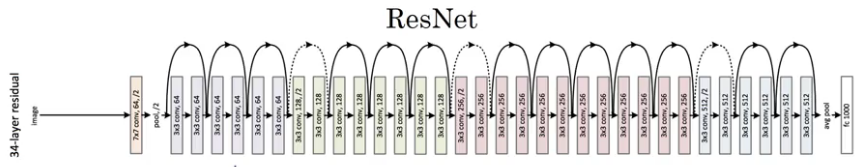### Networks in Networks and 1×1 Convolutions

Let’s see how a 1 X 1 convolution can be helpful. Suppose we have a 28 X 28 X 192 input and we apply a 1 X 1 convolution using 32 filters. So, the output will be 28 X 28 X 32: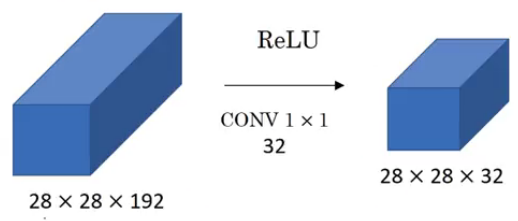The basic idea of using 1 X 1 convolution is to reduce the number of channels from the image. A couple of points to keep in mind:

• We generally use a pooling layer to shrink the height and width of the image
• To reduce the number of channels from an image, we convolve it using a 1 X 1 filter (hence reducing the computation cost as well)

### The Motivation Behind Inception Networks

While designing a convolutional neural network, we have to decide the filter size. Should it be a 1 X 1 filter, or a 3 X 3 filter, or a 5 X 5? Inception does all of that for us! Let’s see how it works.

Suppose we have a 28 X 28 X 192 input volume. Instead of choosing what filter size to use, or whether to use convolution layer or pooling layer, inception uses all of them and stacks all the outputs: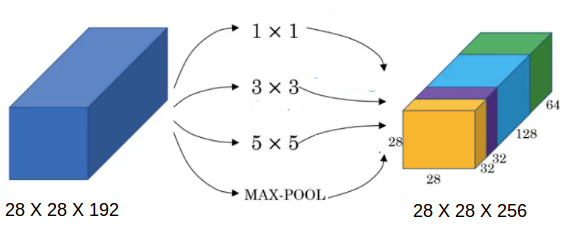A good question to ask here – why are we using all these filters instead of using just a single filter size, say 5 X 5? Let’s look at how many computations would arise if we would have used only a 5 X 5 filter on our input: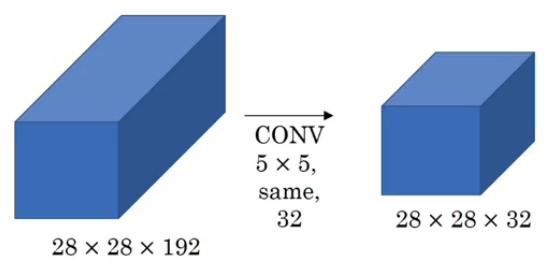Number of multiplies = 28 * 28 * 32 * 5 * 5 * 192 = 120 million! Can you imagine how expensive performing all of these will be?

Now, let’s look at the computations a 1 X 1 convolution and then a 5 X 5 convolution will give us: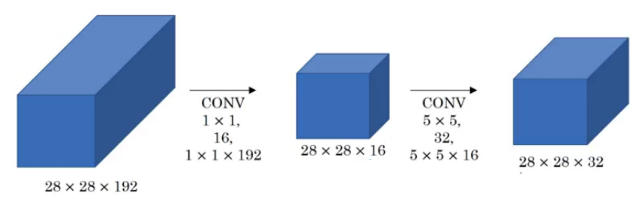Number of multiplies for first convolution = 28 * 28 * 16 * 1 * 1 * 192 = 2.4 million
Number of multiplies for second convolution = 28 * 28 * 32 * 5 * 5 * 16 = 10 million
Total number of multiplies = 12.4 million

A significant reduction. This is the key idea behind inception.

### Inception Networks

This is how an inception block looks: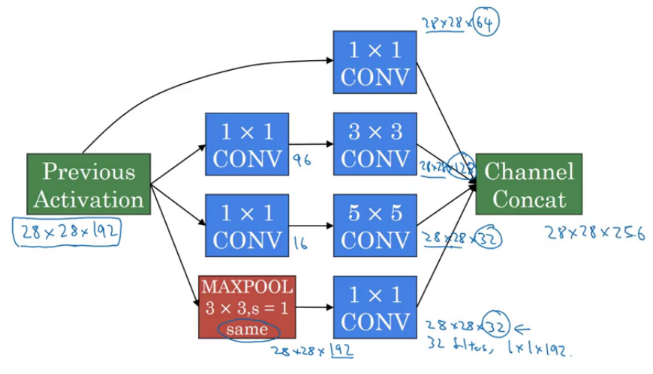We stack all the outputs together. Also, we apply a 1 X 1 convolution before applying 3 X 3 and 5 X 5 convolutions in order to reduce the computations. An inception model is the combination of these inception blocks repeated at different locations, some fully connected layer at the end, and a softmax classifier to output the classes.

Now that we have understood how different ConvNets work, it’s important to gain a practical perspective around all of this.

### Practical advice for using ConvNets

Building your own model from scratch can be a tedious and cumbersome process. Also, it is quite a task to reproduce a research paper on your own (trust me, I am speaking from experience!). In many cases, we also face issues like lack of data availability, etc. We can design a pretty decent model by simply following the below tips and tricks:

1. Using Open-Source implementation: Generally, most deep learning researchers open-source their work on platforms like GitHub. We can (and should) integrate their work into our projects. This has always been a helpful path for me throughout my career
2. Transfer Learning: We can take a pretrained network and transfer that to a new task which we are working on. In transfer learning, we take the complete network, remove a few layers from it, and add custom layers on top of the remaining layers to train our model. In essense, we are extracting features from a pretrained model and using those to classify and train our model
3. Data Augmentation: Deep learning models perform well when we have a large amount of data. There are quite a few domains where getting enough data is a problem. In such cases, we use data augmentation to generate training data from the available data. Some of the common augmentation methods are:
1. Mirroring: Here we take the mirror image. The class of the image will not change in this case
2. Random Cropping
3. Rotating
4. Shearing
5. Color Shifting: We change the RGB scale of the image randomly.

With this, we come to the end of the second module. We saw some classical ConvNets, their structure and gained valuable practical tips on how to use these networks.

## Module 3: Object Detection

The objectives behind the third module are:

• To understand the challenges of Object Localization, Object Detection and Landmark Finding
• Understanding and implementing non-max suppression
• Understanding and implementing intersection over union
• To understand how we label a dataset for an object detection application
• To learn the vocabulary used in object detection (landmark, anchor, bounding box, grid, etc.)

I have covered most of the concepts in this comprehensive article. I highly recommend going through it to learn the concepts of YOLO. For your reference, I’ll summarize how YOLO works:

1. YOLO first takes an input image
2. The framework then divides the input image into grids
3. Image classification and localization are applied on each grid
4. YOLO then predicts the bounding boxes and their corresponding class probabilities for objects

It also applies Intersection over Union (IoU) and Non-Max Suppression to generate more accurate bounding boxes and minimize the chance of the same object being detected multiple times.

In the final module of this course, we will look at some special applications of CNNs, such as face recognition and neural style transfer.

## Module 4: Special Applications: Face Recognition & Neural Style transfer

The objective behind the final module is to discover how CNNs can be applied to multiple fields, including art generation and facial recognition.

### Part 1: Face Recognition

Face recognition is probably the most widely used application in computer vision. It seems to be everywhere I look these days – from my own smartphone to airport lounges, it’s becoming an integral part of our daily activities.

In this section, we will discuss various concepts of face recognition, like one-shot learning, siamese network, and many more.

### What is face recognition?

In face recognition literature, there are majorly two terminologies which are discussed the most:

1. Face verification
2. Face recognition

In face verification, we pass the image and its corresponding name or ID as the input. For a new image, we want our model to verify whether the image is that of the claimed person. This is also called one-to-one mapping where we just want to know if the image is of the same person.

Face recognition is where we have a database of a certain number of people with their facial images and corresponding IDs. When our model gets a new image, it has to match the input image with all the images available in the database and return an ID. It is a one-to-k mapping (k being the number of people) where we compare an input image with all the k people present in the database.

### One-Shot Learning

One potential obstacle we usually encounter in a face recognition task is the problem a lack of training data. This is where we have only a single image of a person’s face and we have to recognize new images using that. Since deep learning isn’t exactly known for working well with one training example, you can imagine how this presents a challenge.

One-shot learning is where we learn to recognize the person from just one example. Training a CNN to learn the representations of a face is not a good idea when we have less images. The model simply would not be able to learn the features of the face. If a new user joins the database, we have to retrain the entire network. Quite a conundrum, isn’t it? So instead of using a ConvNet, we try to learn a similarity function:

d(img1,img2) = degree of difference between images

We train a neural network to learn a function that takes two images as input and outputs the degree of difference between these two images. So, if two images are of the same person, the output will be a small number, and vice versa. We can define a threshold and if the degree is less than that threshold, we can safely say that the images are of the same person.

### Siamese Network

We will use a Siamese network to learn the function which we defined earlier:

d(img1,img2) = degree of difference between images

Suppose we have two images, x(1) and x(2), and we pass both of them to the same ConvNet. Instead of generating the classes for these images, we extract the features by removing the final softmax layer. So, the last layer will be a fully connected layer having, say 128 neurons: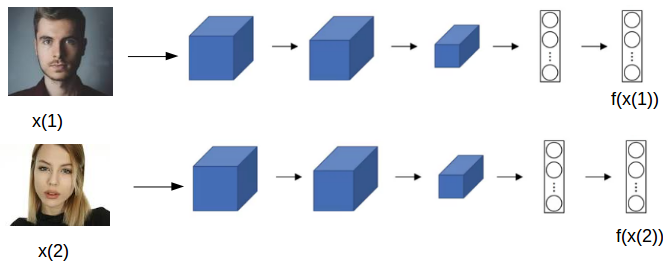Here, f(x(1)) and f(x(2)) are the encodings of images x(1) and x(2) respectively. So,

d(x(1),x(2)) = || f(x(1)) – f(x(2)) ||22

We train the model in such a way that if x(i) and x(j) are images of the same person, || f(x(i)) – f(x(j)) ||2 will be small and if x(i) and x(j) are images of different people, || f(x(i)) – f(x(j)) ||2 will be large. This is the architecture of a Siamese network.

Next up, we will learn the loss function that we should use to improve a model’s performance.

### Triplet Loss

In order to define a triplet loss, we take an anchor image, a positive image and a negative image. A positive image is the image of the same person that’s present in the anchor image, while a negative image is the image of a different person. Since we are looking at three images at the same time, it’s called a triplet loss. We will use ‘A’ for anchor image, ‘P’ for positive image and ‘N’ for negative image.

So, for given A, P and N, we want:

|| f(A) – f(P) ||2  <= || f(A) – f(N) ||2
|| f(A) – f(P) ||2 – || f(A) – f(N) ||2 <= 0

If the model outputs zero for both || f(A) – f(P) ||2 and || f(A) – f(N) ||2, the above equation will be satisfied. The model might be trained in a way such that both the terms are always 0. This will inevitably affect the performance of the model. How do we overcome this? We need to slightly modify the above equation and add a term 𝛼, also known as the margin:

|| f(A) – f(P) ||2 – || f(A) – f(N) ||2 + 𝛼 <= 0

The loss function can thus be defined as:

L(A,P,N) = max(|| f(A) – f(P) ||2 – || f(A) – f(N) ||2 + 𝛼, 0)

Similarly, the cost function for a set of people can be defined as:Our aim is to minimize this cost function in order to improve our model’s performance. Apart with using triplet loss, we can treat face recognition as a binary classification problem.

### Face Verification and Binary Classification

Instead of using triplet loss to learn the parameters and recognize faces, we can solve it by translating our problem into a binary classification one. We first use a Siamese network to compute the embeddings for the images and then pass these embeddings to a logistic regression, where the target will be 1 if both the embeddings are of the same person and 0 if they are of different people: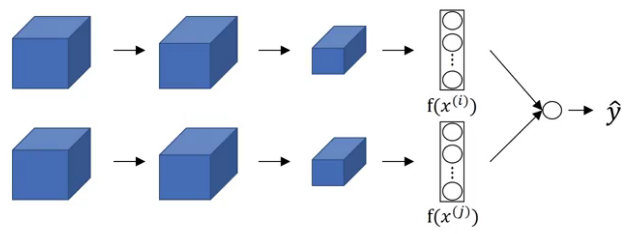The final output of the logistic regression is: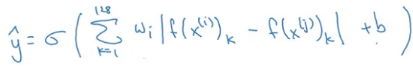Here, 𝜎 is the sigmoid function. Hence, we treat it as a supervised learning problem and pass different sets of combinations. Each combination can have two images with their corresponding target being 1 if both images are of the same person and 0 if they are of different people.

### Part 2: Neural Style Transfer

In the final section of this course, we’ll discuss a very intriguing application of computer vision, i.e., neural style transfer.

### What is neural style transfer?

Let’s understand the concept of neural style transfer using a simple example. Suppose we want to recreate a given image in the style of another image. Here, the input image is called as the content image while the image in which we want our input to be recreated is known as the style image: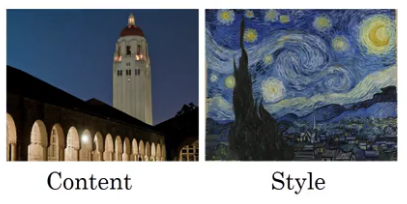Neural style transfer allows us to create a new image which is the content image drawn in the fashion of the style image: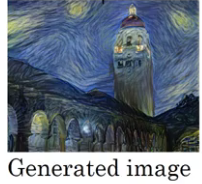Awesome, right?! For the sake of this article, we will be denoting the content image as ‘C’, the style image as ‘S’ and the generated image as ‘G’. In order to perform neural style transfer, we’ll need to extract features from different layers of our ConvNet.

### What are deep ConvNets learning?

Before diving deeper into neural style transfer, let’s first visually understand what the deeper layers of a ConvNet are really doing. Let’s say we’ve trained a convolution neural network on a 224 X 224 X 3 input image: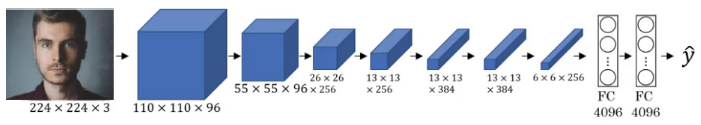To visualize each hidden layer of the network, we first pick a unit in layer 1, find 9 patches that maximize the activations of that unit, and repeat it for other units. The first hidden layer looks for relatively simpler features, such as edges, or a particular shade of color. The image compresses as we go deeper into the network. The hidden unit of a CNN’s deeper layer looks at a larger region of the image.

As we move deeper, the model learns complex relations: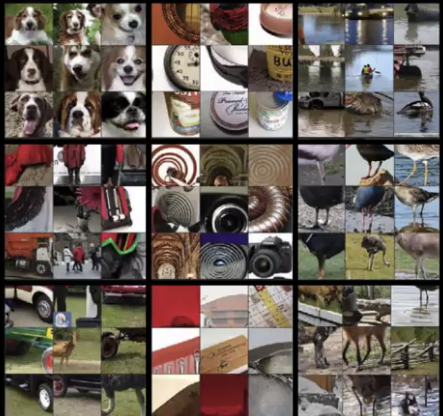This is what the shallow and deeper layers of a CNN are computing. We will use this learning to build a neural style transfer algorithm.

### Cost Function

First, let’s look at the cost function needed to build a neural style transfer algorithm. Minimizing this cost function will help in getting a better generated image (G). Defining a cost function:

J(G) = ⍺*JContent(C,G) + β*JStyle(S,G)

Here, the content cost function ensures that the generated image has the same content as that of the content image whereas  the generated cost function is tasked with making sure that the generated image is of the style image fashion.

Below are the steps for generating the image using the content and style images:

1. We first initialize G randomly, say G: 100 X 100 X 3, or any other dimension that we want
2. We then define the cost function J(G) and use gradient descent to minimize J(G) to update G:
G = G – d/dG(J(G))

Suppose the content and style images we have are: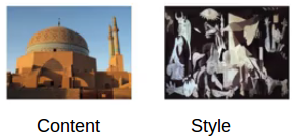First, we initialize the generated image: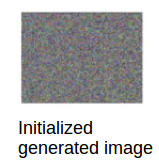After applying gradient descent and updating G multiple times, we get something like this: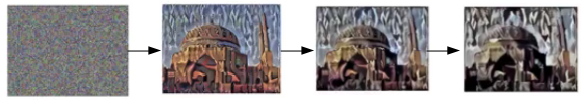Not bad! This is the outline of a neural style transfer algorithm. It’s important to understand both the content cost function and the style cost function in detail for maximizing our algorithm’s output.

### Content Cost Function

Suppose we use the lth layer to define the content cost function of a neural style transfer algorithm. Generally, the layer which is neither too shallow nor too deep is chosen as the lth layer for the content cost function. We use a pretrained ConvNet and take the activations of its lth layer for both the content image as well as the generated image and compare how similar their content is. With me so far?

Now, we compare the activations of the lth layer. For the content and generated images, these are a[l](C) and a[l](G) respectively. If both these activations are similar, we can say that the images have similar content. Thus, the cost function can be defined as follows:

JContent(C,G) = ½ * || a[l](C) – a[l](G) ||2

We try to minimize this cost function and update the activations in order to get similar content. Next, we will define the style cost function to make sure that the style of the generated image is similar to the style image.

### Style Cost Function

Suppose we pass an image to a pretrained ConvNet: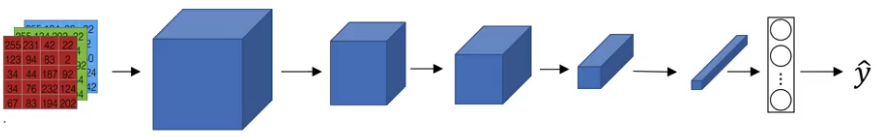We take the activations from the lth layer to measure the style. We define the style as the correlation between activations across channels of that layer. Let’s say that the lth layer looks like this: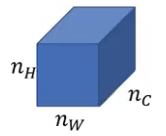We want to know how correlated the activations are across different channels:

ai,j,k = activations at (i,j,k)

Here, i is the height, j is the width, and k is the channel number. We can create a correlation matrix which provides a clear picture of the correlation between the activations from every channel of the lth layer: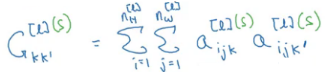where k and k’ ranges from 1 to nc[l]. This matrix is called a style matrix. If the activations are correlated, Gkk’ will be large, and vice versa. S denotes that this matrix is for the style image. Similarly, we can create a style matrix for the generated image: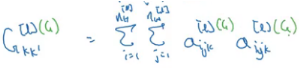Using these two matrices, we define a style cost function: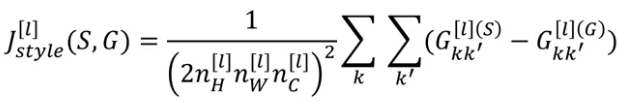This style cost function is for a single layer. We can generalize it for all the layers of the network: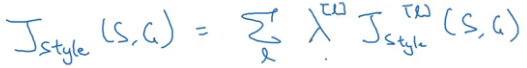Finally, we can combine the content and style cost function to get the overall cost function:

J(G) = ⍺*JContent(C,G) + β*JStyle(S,G)

And there you go! Quite a ride through the world of CNNs, wasn’t it?

## End Notes

We have learned a lot about CNNs in this article (far more than I did in any one place!). We have seen how a ConvNet works, the various building blocks of a ConvNet, it’s various architectures and how they can be used for image recognition applications. Finally, we have also learned how YOLO can be used for detecting objects in an image before diving into two really fascinating applications of computer vision – face recognition and neural style transfer.

Do share your throughts with me regarding what you learned from this article. Have you used CNNs before? If yes, feel free to share your experience with me – it always helps to learn from each other.###### Pulkit Sharma

My research interests lies in the field of Machine Learning and Deep Learning. Possess an enthusiasm for learning new skills and technologies.

## 8 thoughts on "A Comprehensive Tutorial to learn Convolutional Neural Networks from Scratch (deeplearning.ai Course #4)"###### Eric.Wilson @MoonMarketing says:December 27, 2018 at 1:37 pm
This post is exceptional. Very rich in information and insights. Truly unique ... Google loves this post ... in fact I found it through search. You satisfied my research intent. thank you so much Eric Wilson @moonmarketing Reply###### Praaksh says:January 17, 2019 at 12:11 pm
The best of article, I have seen so far regarding CNN, not too deep and not too less. Just the right mixture to get an good idea on CNN, the architecture. I wish if there was GitHub examples posted for all the above use cases (Style Transfer, SSD etc.) Reply###### Pulkit Sharma says:January 17, 2019 at 3:34 pm
Hi Praaksh, Glad that you liked the article! You can get the codes here. Reply###### AKhila says:January 18, 2019 at 10:12 am
Very Informative. Can you please share link to Course 3. Structuring Machine Learning Projects & Course 5. Sequence Models. Thanks. Reply###### Pulkit Sharma says:January 18, 2019 at 11:15 am